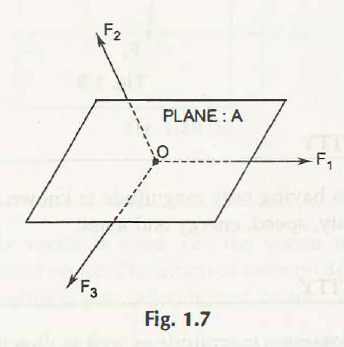Coplanar means the forces act on the same plane. So coplanar concurrent means the forces on the same place act on the same point. It can be explained in terms of three different forces in which F1, F2 and F3 meet at the common point known as O.So, this system of force is known as coplanar concurrent forces of the system. Intersecting at the single point is known as concurrent forces. Here the plane is A. A number of work can be done by applying this where the various forces must have a single point to apply. This is also important to understand through the different processes and thus analytical as well as graphical are the perfect way of determining this. In case of the different points of intersecting in a graphical representation, then resultant would not be considered as the coplanar concurrent. So, investigations must be done in a proper way.

Links of Next Mechanical Engineering Topics:-### Customer Reviews

My Homework Help
Rated 5.0 out of 5 based on 510 customer reviews at